# Mapping the fourfold H4 600-cells emerging from E8: A mathematical and visual study

My latest paper is now available here or on Vixra.

{abstract}
It is widely known that the E8 polytope can be folded into two Golden Ratio (Φ) scaled copies of the 4 dimensional (4D) 120 vertex 720 edge H4 600-cell. While folding an 8D object into a 4D one is done by applying the dot product of each vertex to a 4×8 folding matrix, we use an 8×8 rotation matrix to produce four 4D copies of H4 600-cells, with the original two left side scaled 4D copies related to the two right side 4D copies in a very specific way. This paper will describe and visualize in detail the specific symmetry relationships which emerge from that rotation of E8 and the emergent fourfold copies of H4. It will also introduce a projection basis using the Icosahedron found within the 8×8 rotation matrix. It will complete the detail for constructing E8 from the 3D Platonic solids, Icosians, and the 4D H4 600-cell. Eight pairs of Φ scaled concentric Platonic solids are identified directly using the sorted and grouped 3D projected vertex norms present within E8. Finally, we will show the relationship of the Beordijk-Coxeter Tetrahelix emerging from the Petrie projection’s concentric rings of 30 vertices of the H4 600-cells.

This paper builds off of some recent work here, and here.

Below are a few figures from that paper.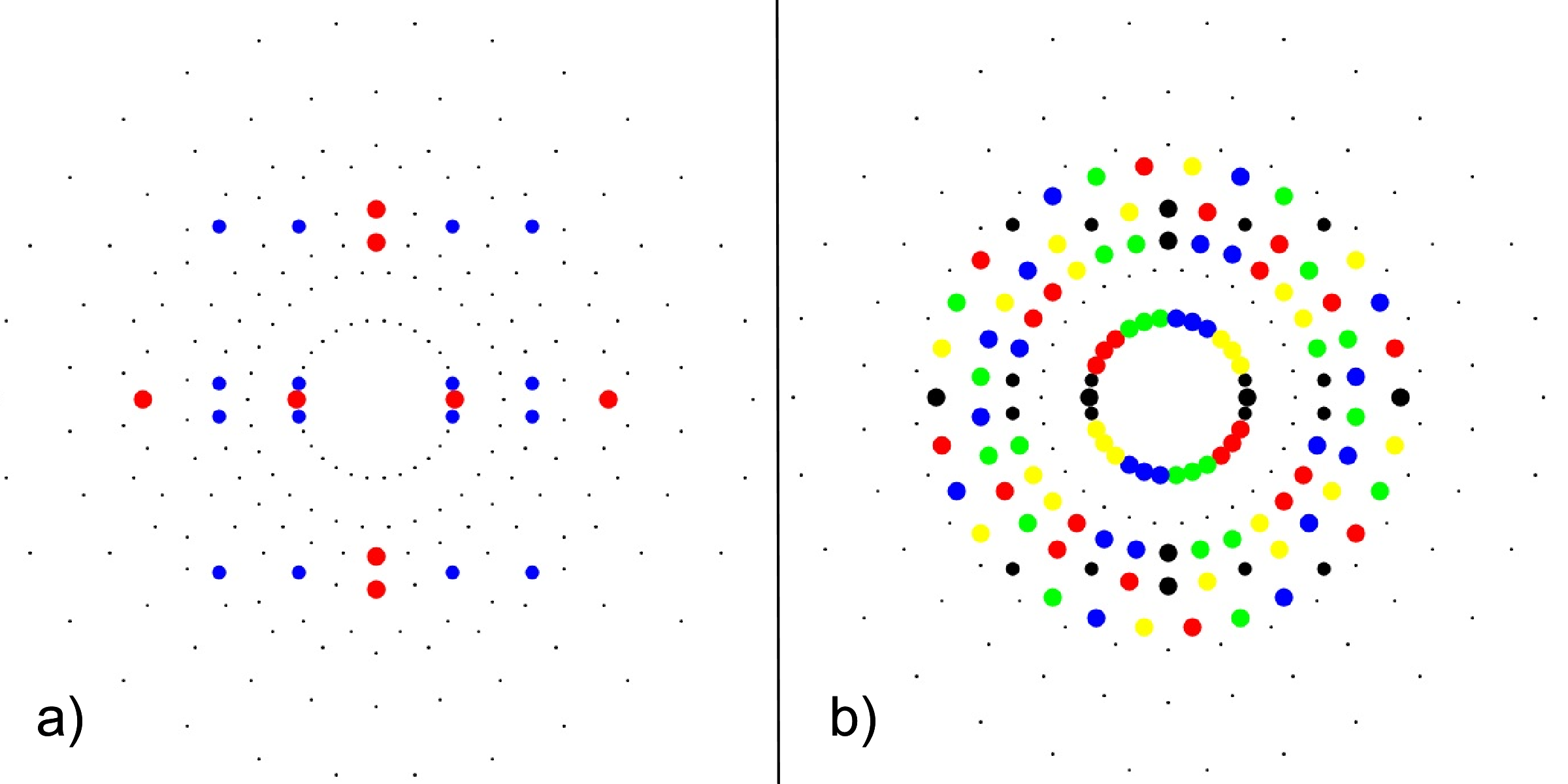FIG. 3: a) 24-cell highlighting the 16-cell (red on-axis vertices) and 8-cell (blue off-axis vertices), b) Snub 24-cell highlighting four pi/5 rotations of the 24-cell (black) in red, green, blue, yellow.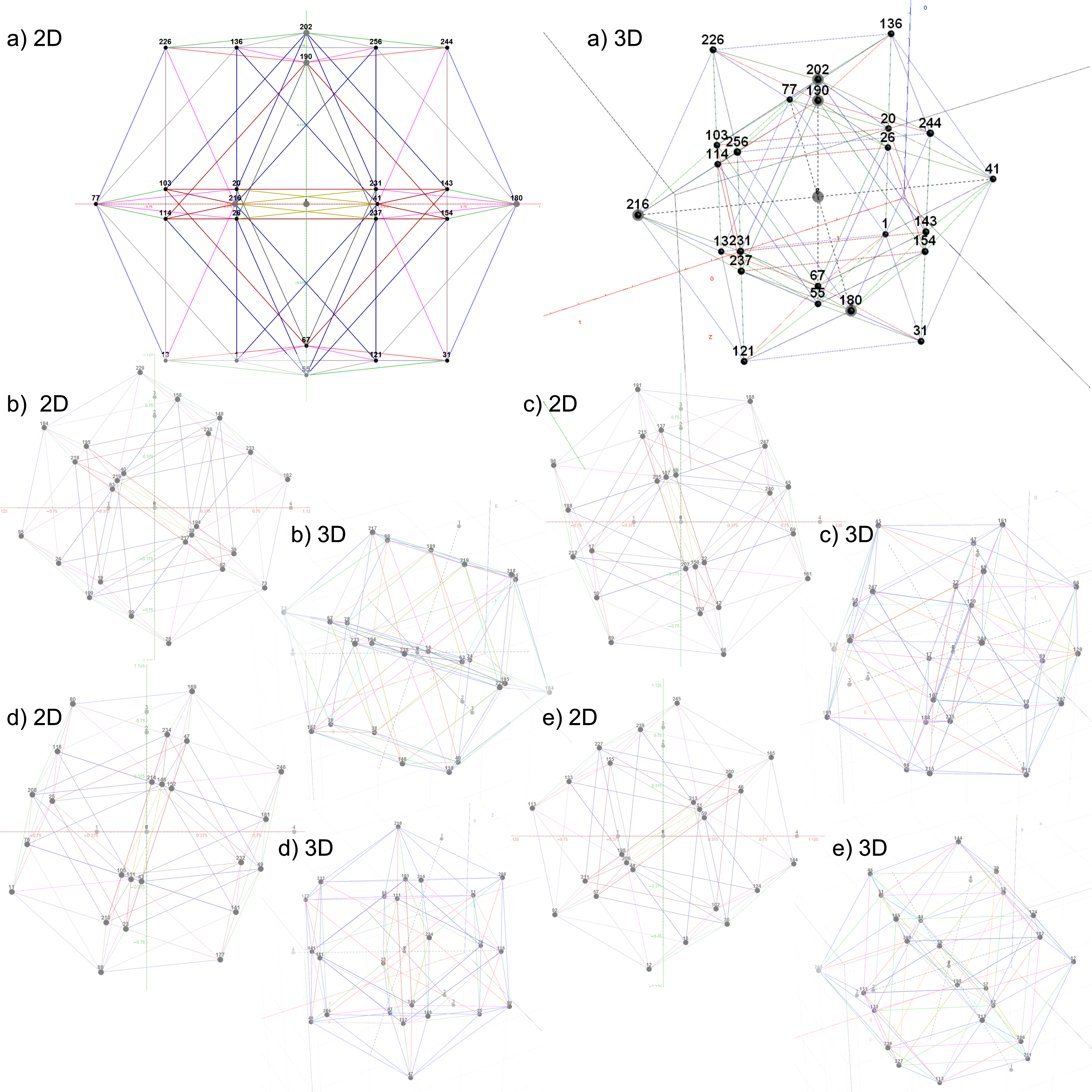FIG. 4: The 5 24-cell decomposition of one 600-cell, each with 2D and 3D vertex numbered projections including edges; a)
24-cell, b-e) Snub 24-cells from four pi/5 rotations of the 24-cell in a)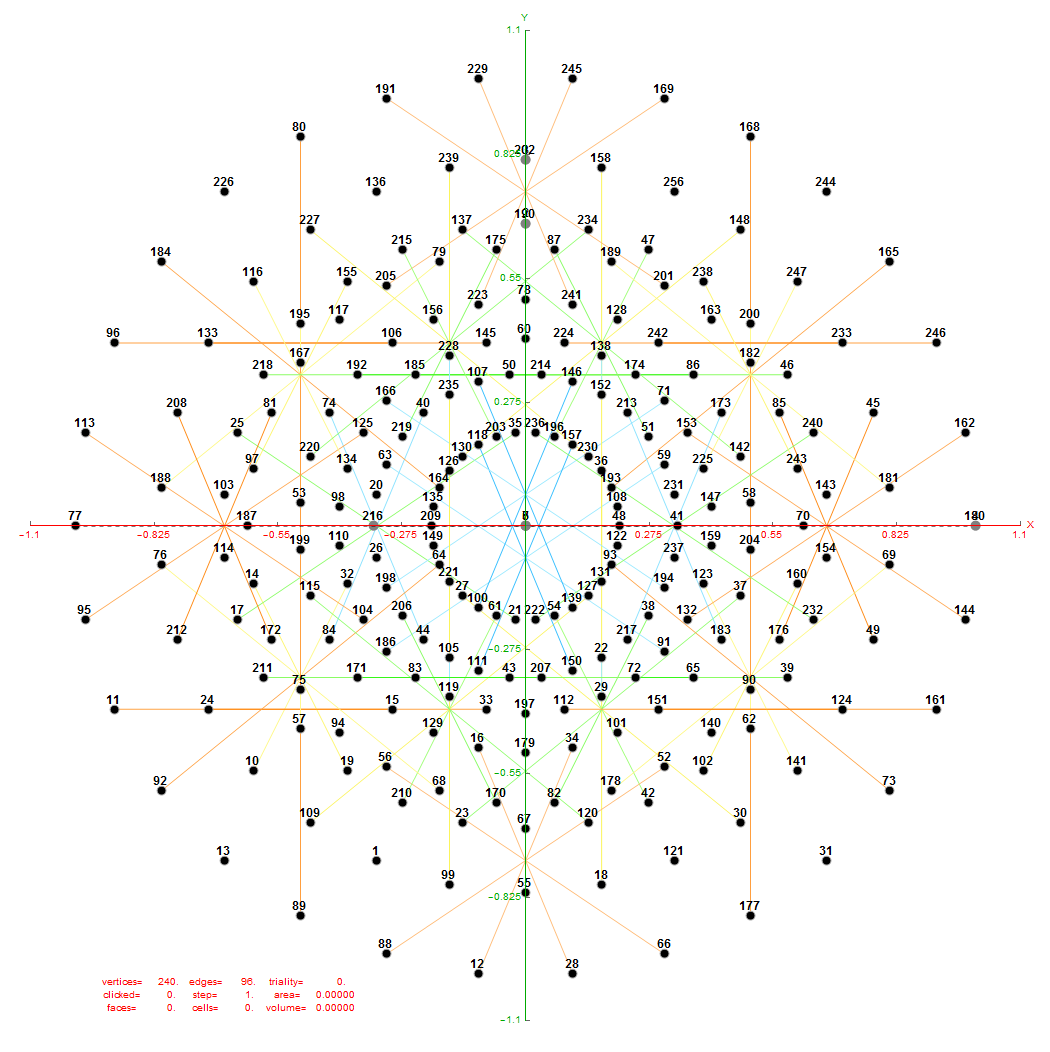FIG. 6: Vertex numbered Petrie projection of the rotated E8, showing the 96 edges of 8D norm l = 2Φ which links the Snub 24-cell H4 and H4Φ (L<->R) vertices (Note: the 8 excluded positive E8 8-Orthoplex (or equivalently, the H4 and H4Φ 4-Orthoplex) “generator vertices” are shown as larger gray labeled axis dots which overlap their darker black E8 vertices)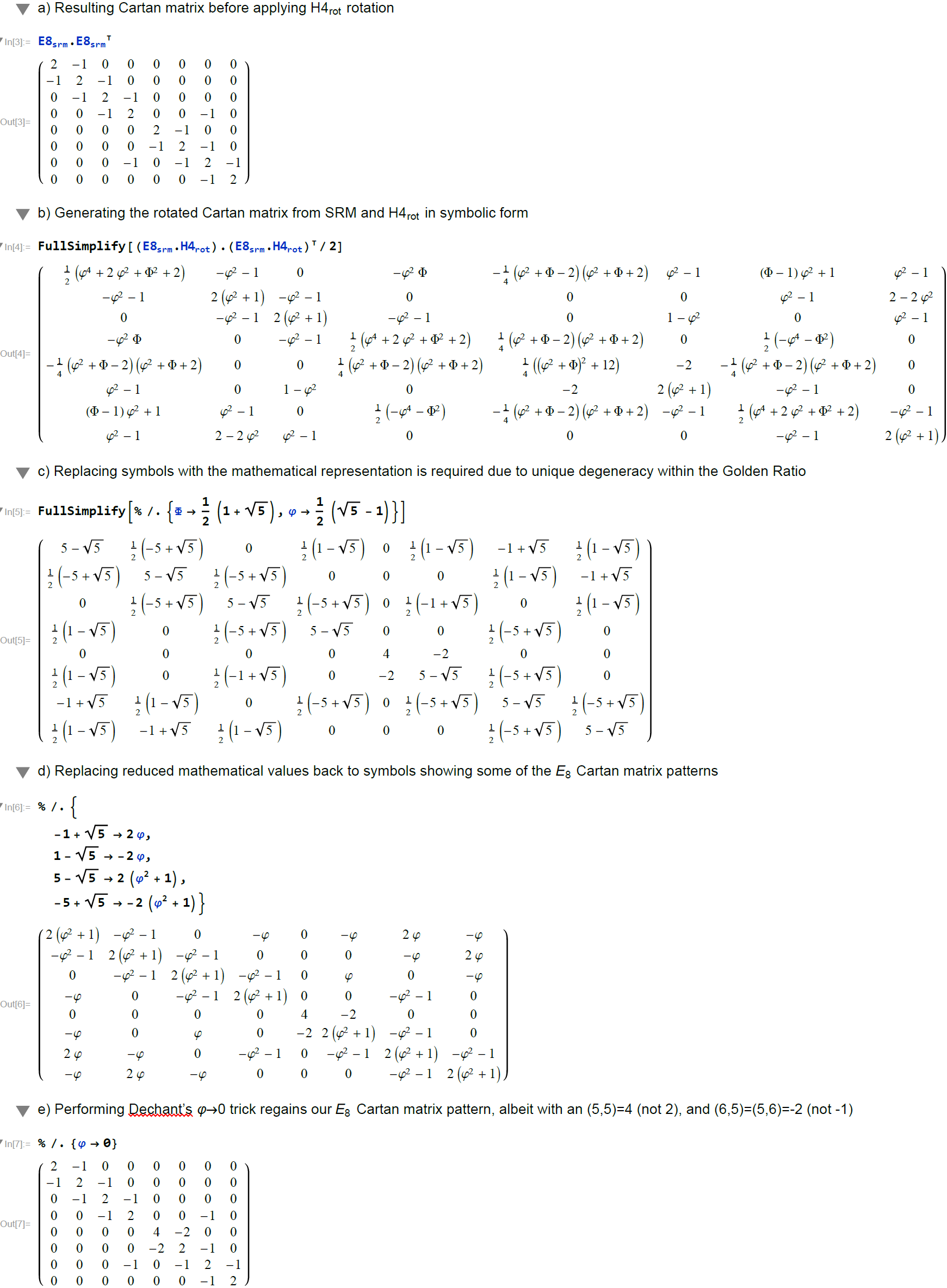FIG. 7: Symbolic analysis using MathematicaTM comparing the Cartan matrix before and after rotating the simple roots matrix (E8srm) used to create it (Note: the resulting Cartan matrix is not precisely that of E8, even after applying the Dechant’s Φ=0 trick)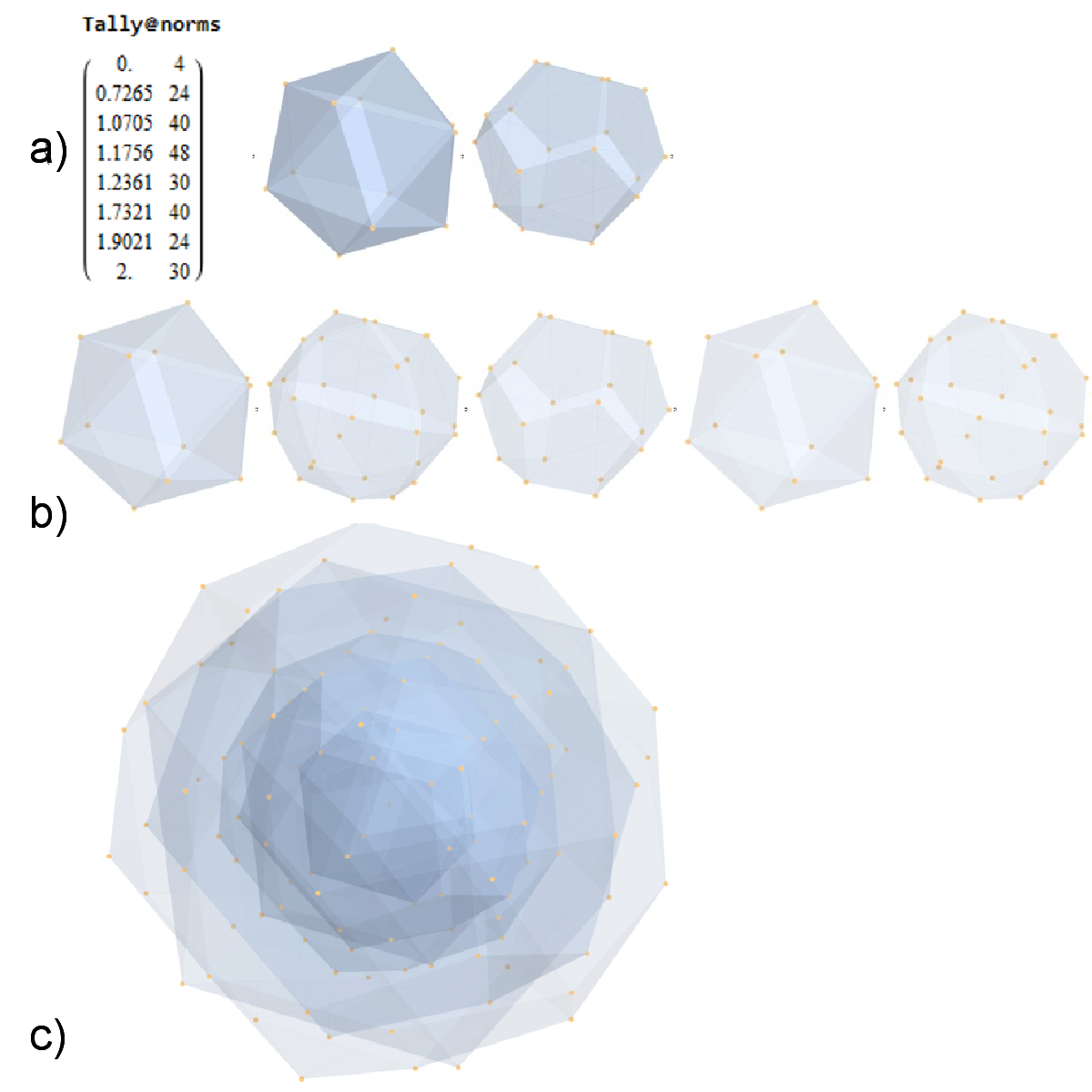FIG. 8: a) Sorted list of the vertex norms with their grouped vertex counts. b) 3D surface models for each of the 7 hulls of vertices c) Combined 3D surface model with increasing transparency for each successive hull.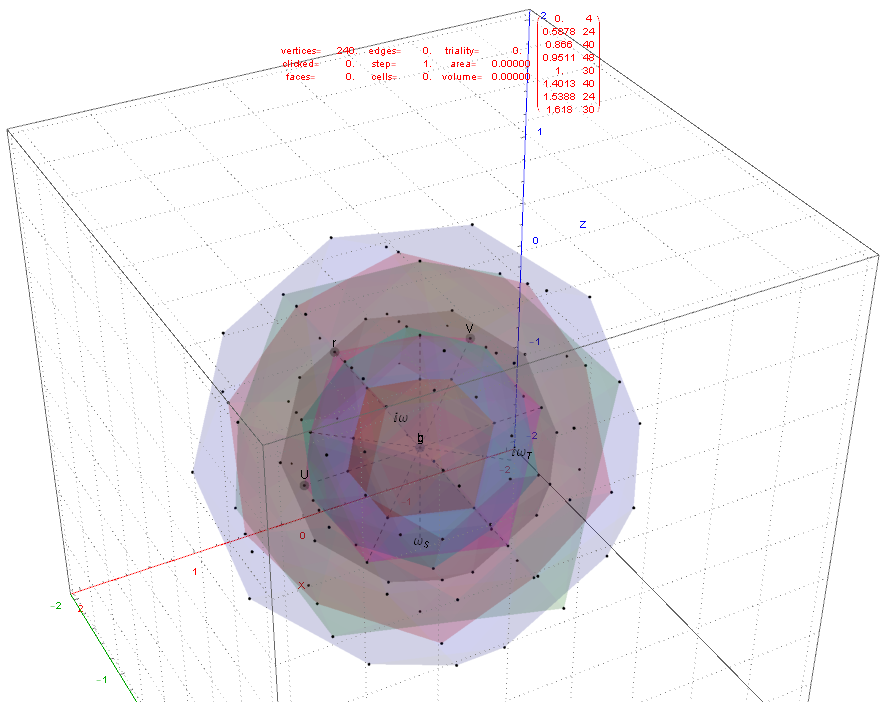FIG. 11: 3D projection using an orthonormal basis on H4 and H4Φ vertices (i.e. the rotated E8)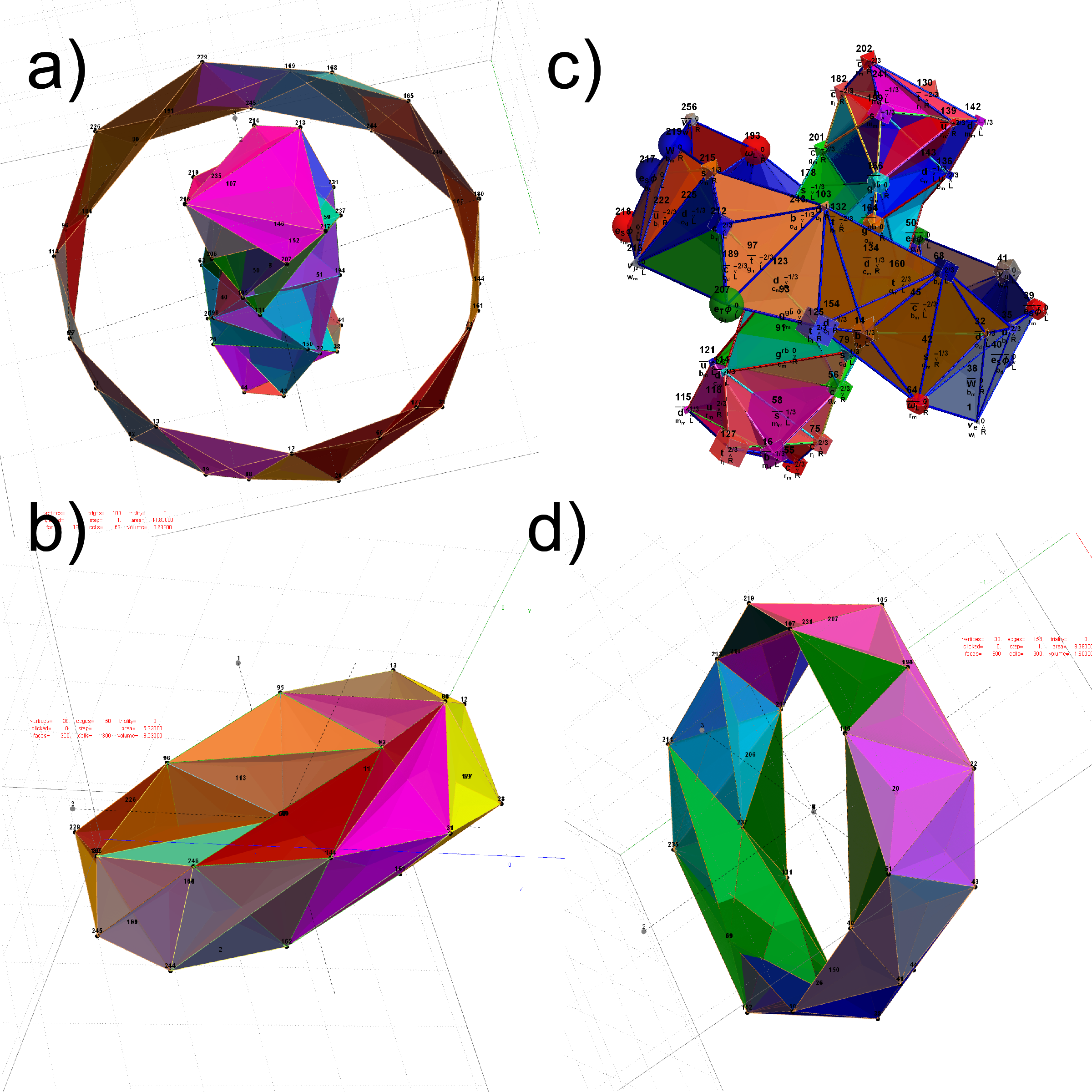FIG. 12: The Beordijk-Coxeter Tetrahelix emerging from the 8 concentric rings of 30 E8 and H4+H4Φ Petrie projection vertices; a) H4 inner and outer ring in 3D, b) with vertex size, shape, color assigned from physics model, c-d) inner and outer ring in orthonormal projection of H4Φ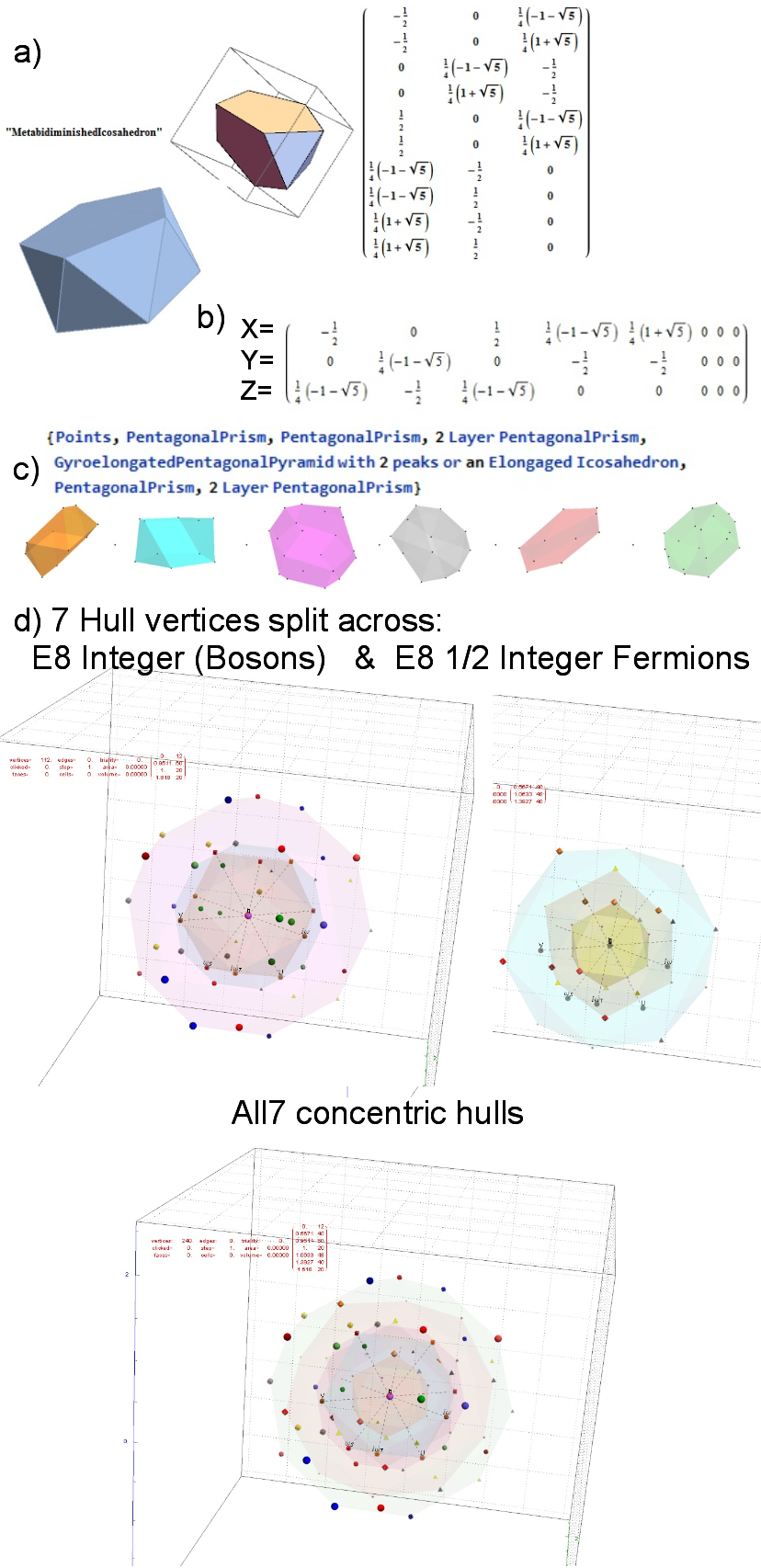FIG. 15: MetabidiminishedIcosahedron (with its negated vertices gives the GyroelongatedPentagonalPyramid) Crystal Projection Prism projecting E8 a) the crystal prism geometry and vertices, b) the selected basis vectors, c) the individual 3D projected concentric hull objects, d) the combined set of concentric objects with progressively increasing transparency and tally of vertex norms.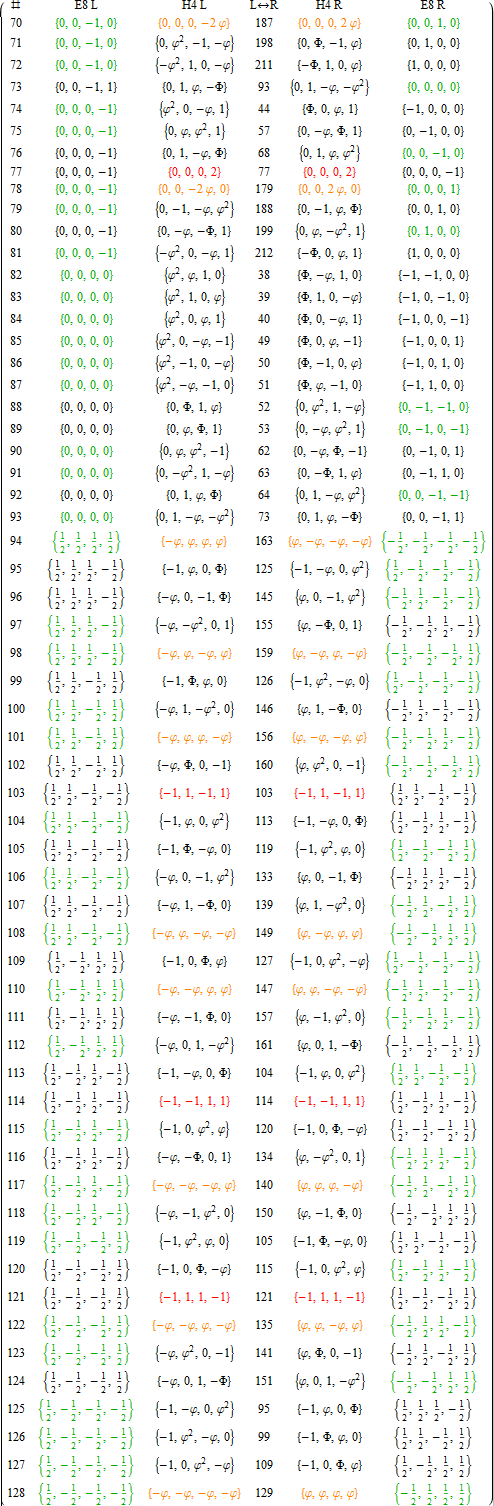APPENDIX A: LIST OF E8 AND ITS ROTATED H4 AND H4Φ VERTICES
This table splits the E8 and its rotated H4 vertices into L/R 4D elements.

1. Table structure
The first column is an SRE E8 vertex index number derived from sorting the E8 vertices by their position based on the 256=2^8 binary pattern from the 9th row of the Pascal triangle (1, 8, 28, 56, 70, 56, 28, 8, 1) and its associated Cl8 Clifford Algebra. This construction is described in more detail in . The odd groups (1,3,5,7,9) with (1,26,70,26,1) elements (respectively) are the 128 1/2 integer vertices. The even groups (2,4,6,8) with (8,56,56,8) elements (respectively) are the 112 integer vertices along with the 16 excluded 8 generator (and 8 anti-generator) vertices (2-9 and 248-255) with permutations of (1; 0; 0; 0; 0; 0; 0; 0) (a.k.a. the 8-Orthoplex), such that they indicate the basis vectors used for projecting the polytope.

Only the first half of the 240 vertices is shown, since the last half is simply the reverse order negation of the vertices
in the first half (e.g. the E8 vertex n=10 has as its’ negation 257-n=247). The middle column labeled L<->R indicates the vertex reference number that contains the same L as the R (and equivalently, the same R as the L, interestingly enough).

2. Table color coding
The E8 L/R columns’ green color-coded elements indicate that the 4D vertex rotates into the smaller H4 600-cell.
Conversely, the E8 L/R columns’ black color-coded elements indicate the 4D vertex rotates into the larger H4Φ
600-cell.

The H4 L/R columns’ red color-coded rows are 24-cell elements that always self-reference and are always members of the scaled up 600-cell H4Φ. The H4 L/R columns’ orange color-coded rows are 24-cell elements that always reference the negated elements in the range of 129-256 and are always members of the smaller 600-cell H4.

# Comparing nearest edges between Split Real Even (SRE) E8

I have been intrigued with Richter’s arXiv 0704.3091 “Triacontagonal Coordinates for the E8 root system” paper.

Comparing nearest edges between Split Real Even (SRE) E8 and Richter’s Complex 4D Golden Ratio Petrie Projection Model:

Please note, the vertex numbers are reordered from Richter’s paper in order to be consistent with ArXiv quant-ph 1502.04350 “Parity Proofs of Kochen-Specker theorem based on the Lie Algebra E8” by Aravind and Waegell. This paper uses the beautiful symmetries of E8 as a basis for proof sets related to the Bell inequalities of quantum mechanics. Below is a graphic showing that vertex number ordering: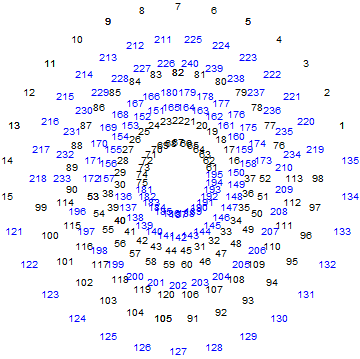Richter’s model with 74 nearest edges per vertex (Complex 4D Norm’d length Sqrt, with 8880 total):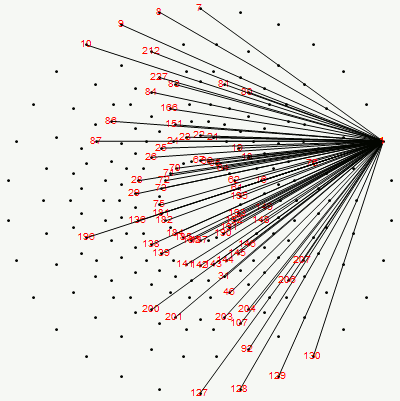Richter’s model with only 26 nearest edges per vertex (Complex 4D Norm’d length 1, 3120 total):The above unity length edge pattern has is more consistent with the SRE E8 below.

The SRE E8 Petrie Projection (with 56 Norm’d edge length Sqrt for each vertex, 6720 total). This is equivalent to folding E8 to H4 with an 8×8 rotation matrix which creates a 4D-left H4+H4*Phi and a 4D-right H4+H4*Phi.

Please note, the vertex number is that of the E8 vertices rotated (or “folded”) to H4 in 2D Petrie projection. The vertex numbers are in canonical binary order from E8’s 1:1 correspondence with the 9th row of the Pascal Triangle (eliminating the 16 generator/anti-generator vertices of E8 found in the 2nd and 8th column of the Pascal Triangle). The graphic below shows the vertex number ordering: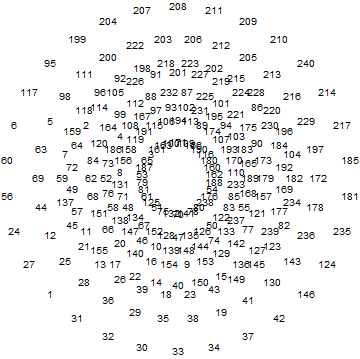Here we take the 4D-left half and project to two concentric rings of H4 and H4 Phi (Golden Ratio) with 56 nearest edges per vertex (Norm’d length of unity or Phi for each vertex):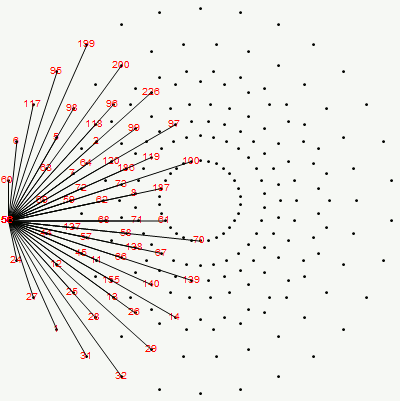The animation frames are sorted by the ArcTan[y/x] of the vertex position in each ring (sorted H4*Phi outer to inner, then H4 outer to inner).

BTW – if you find this information useful, or provide any portion of it to others, PLEASE make sure you cite this post. If you feel a blog post citation would not be an acceptable form for academic research papers, I would be glad to clean it up and put it into LaTex format in order to provide it to arXiv (with your academic sponsorship) or Vixra. Just send me a note at:  jgmoxness@theoryofeverthing.org.

# Universal Time’s Arrow as a Broken Symmetry of an 8D Crystallographic E8 folding to self dual H4+H4φ 4D QuasiCrystal spacetime

Animations of Wolfram’s Cellular Automaton rule 224 in a 3D version of Conway’s Game of Life.

While this does not yet incorporate the E8 folding to 4D H4+H4φ construct, the notion of applying it, as the title suggests, to a fundamental particle physics simulation of a “Quantum Computational Universe” or an emergent (non)crystallographic genetic DNA (aka. Life) is an interesting thought…

Watch a few notional movies, some in left-right stereo 3D. Best viewed in HD mode.

These are snapshots of all the different initial conditions on the rule 224. Each set of 25 has a different object style and/or color gradient selection.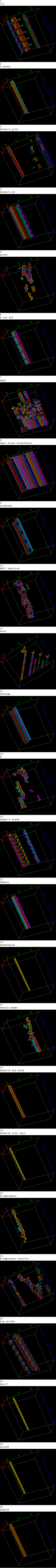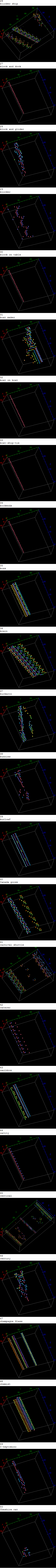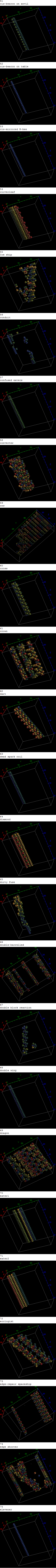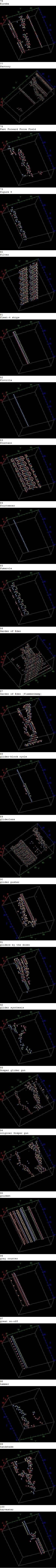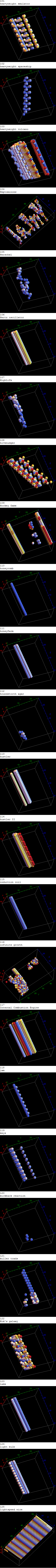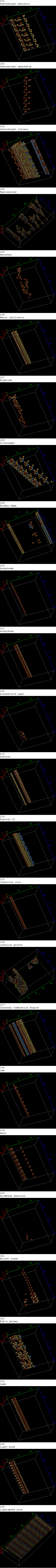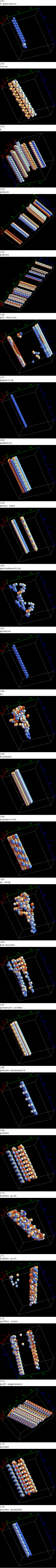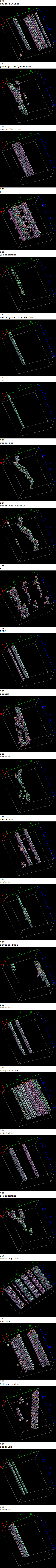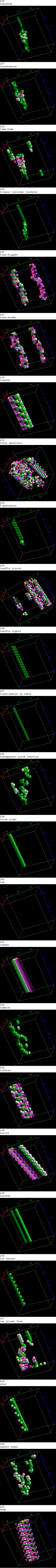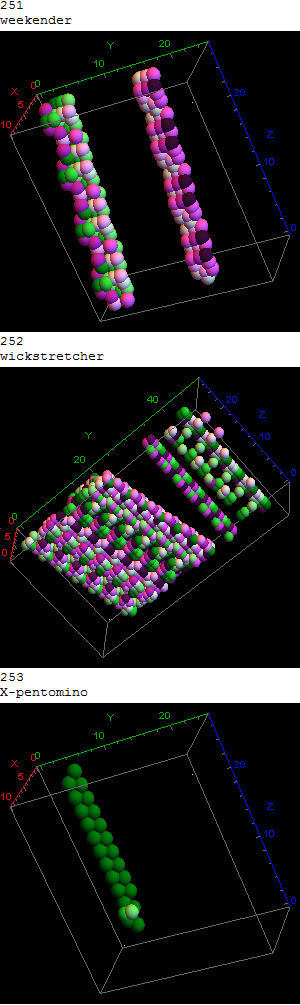# Playing around visualizing non-crystallographic DNA/RNA

I’ve got interaction between the DNA/RNA protein visualizations (e.g. “1F8V- PARIACOTO VIRUS REVEALS A DODECAHEDRAL CAGE OF DUPLEX RNA) with the VisibLie_E8 projections of crystallographic E8 to non-crystallographic H4 (and to dodecahedral H3 in 3 dimensions, of course).

These pics are a simple (naive) merge of the D6 projected using the E8 to H4 folding matrix and the Protein DB at http://www.rcsb.org/ for 1F8V).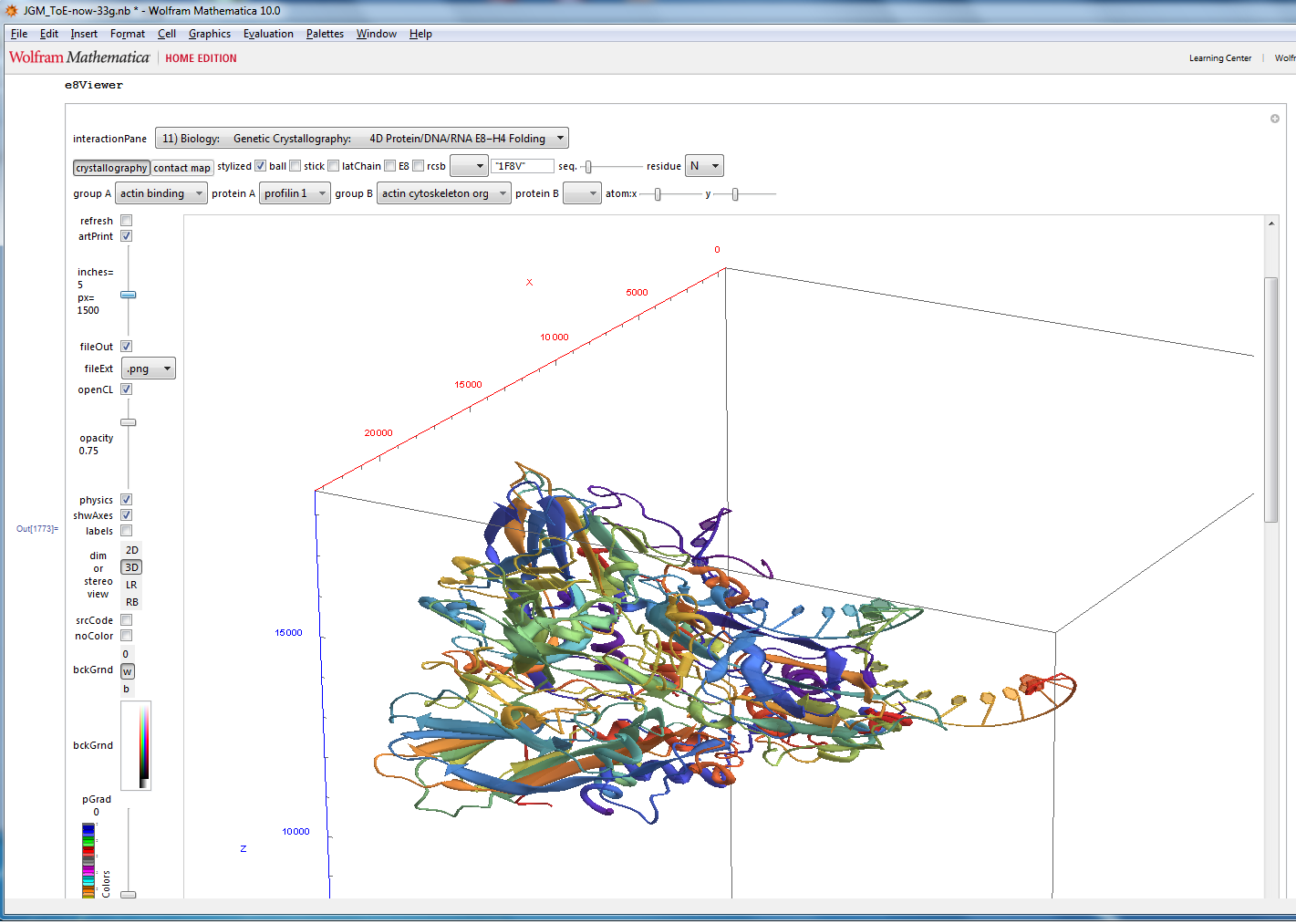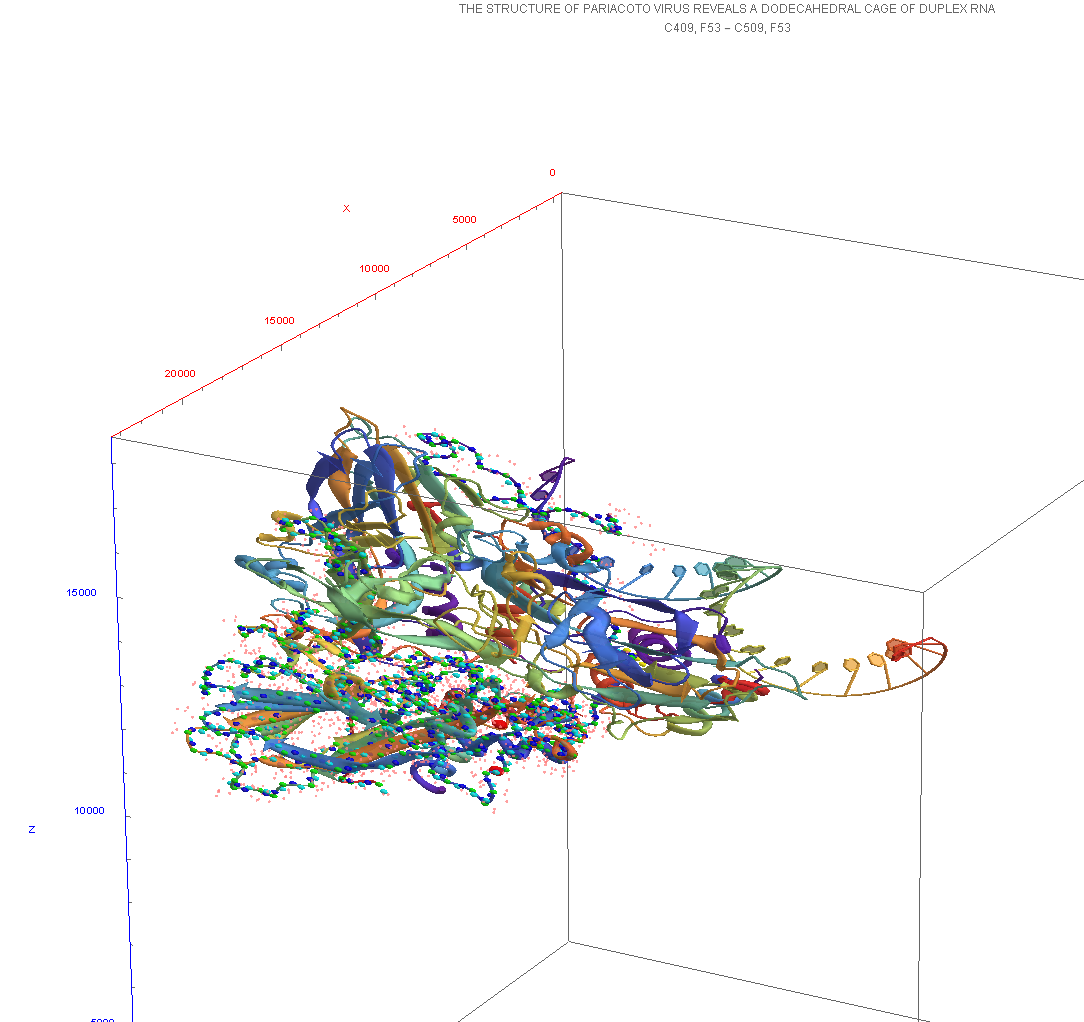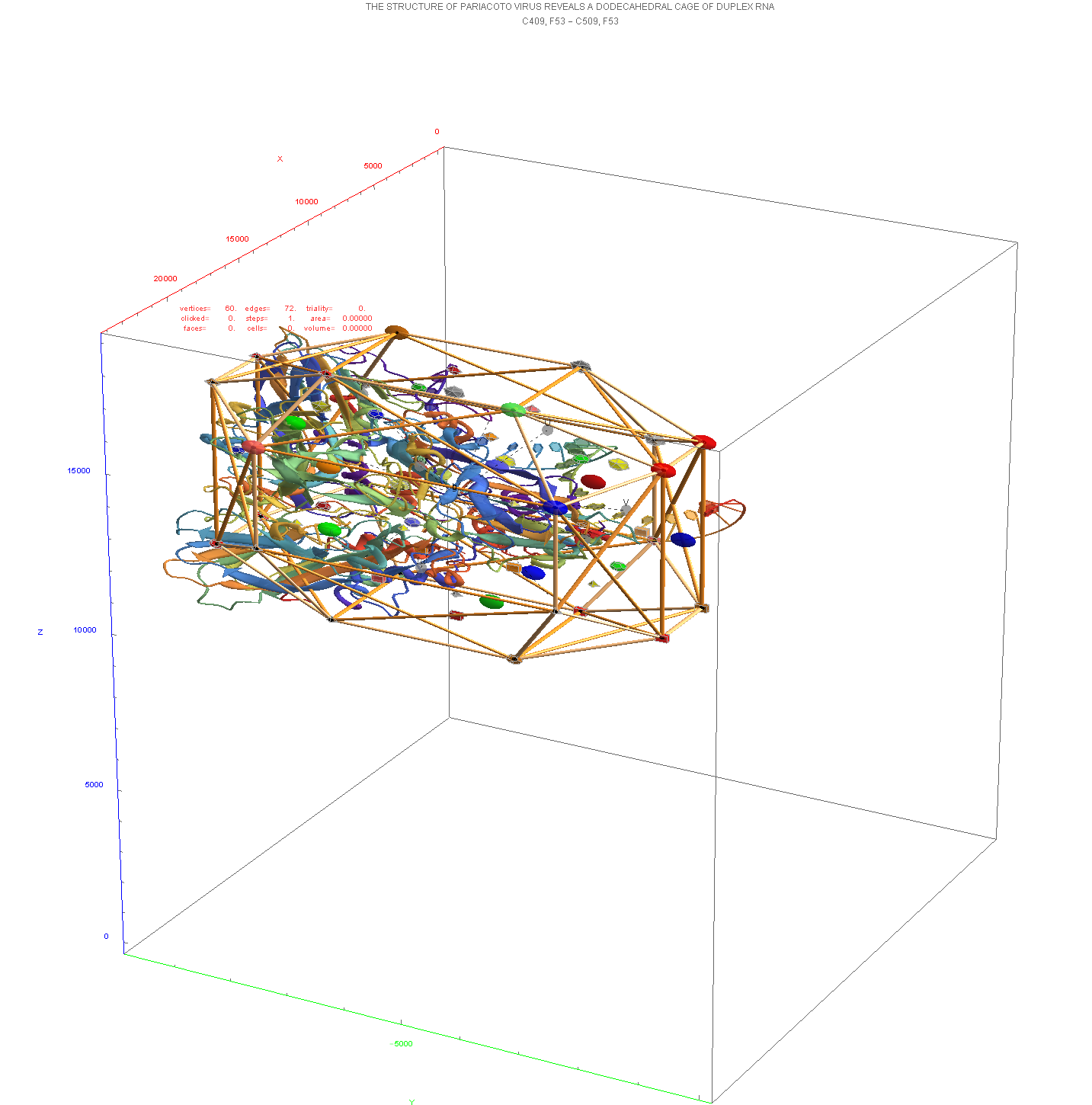# D6 projected to 3D using the E8 to H4 Folding Matrix

This blogs.ams.org/visualinsight article is an American Mathematical Society post based on some of my work. I’ve made an animation of the D6 polytope with 60 vertices and 480 edges of 6D length Sqrt(2). It is similar to the one in the article by Greg Egan. This version was made from my VisibLie E8 demonstration software.

Best viewed in HD mode.

The process for making this using the .nb version of VisibLie E8 ToE demonstration:
1) Side menu selections:

• slide “inches” to 4 (lowers the px resolution)
• select “3D”
• set bckGrnd “b” (black)
• uncheck the shwAxes box
• check the physics box (to give it interesting vertex shapes/colors)
• change the fileExt to “.avi” and check the fileOut box (to save the .avi)
• check the artPrint box (to give it cylinder-like edges)

2) Go to the #9) HMI nD Human Interface pane:
2a) Top menu selections:

• set pScale to .08 (to increase the vertex shape sizes)

2b) Inside the pane selections:

• set “eRadius” to .01 (for thicker edge lines)

3) Go to the #2) Dynkin pane and interactively create (or select from the drop down menu) the D6 diagram.
BTW – this actually selects the subset of vertices from the E8 polytope that are in D6.

4) Go to the #3) E8 Lie Algebra pane:
4a) Top menu selections:

• E8->H4 projection
• check the edges box
• “spin” animation
• 30 animation “steps”

or simply upload this file or copy / paste the following into a file with extension of “.m” and select it from the “input file” button on the #9 HMI nD Human Interface pane.

(* This is an auto generated list from e8Flyer.nb *)
new :={
artPrint=True;
inches=4;
physics=True;
p3D=” 3D”;
bckGrnd=GrayLevel;
fileExt=”.avi”;
shwAxes=False;
scale=0.08;
selPrj=”E8->H4″;
lie=”D6″;
cylR=0.01;
showEdges=True;
steps=30;
pthRot=”Spin”;
selPrj=”E8->H4″;
p3D=” 3D”;
};new;

In the next version I will just make this another “MetaFavorite”, so it will be in the dropdown menu in all versions.
Enjoy!